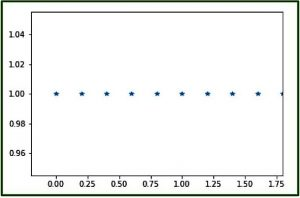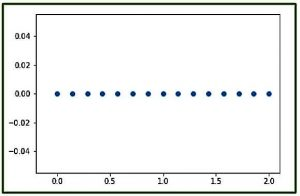# numpy.linspace() in Python

The numpy.linspace() function returns number spaces evenly w.r.t interval. Similar to numpy.arange() function but instead of step it uses sample number.
Syntax :

```numpy.linspace(start,
stop,
num = 50,
endpoint = True,
retstep = False,
dtype = None)```

Parameters :

```-> start  : [optional] start of interval range. By default start = 0
-> stop   : end of interval range
-> restep : If True, return (samples, step). By deflut restep = False
-> num    : [int, optional] No. of samples to generate
-> dtype  : type of output array
```

Return :

```-> ndarray
-> step : [float, optional], if restep = True
```

Code 1 : Explaining linspace function

 `# Python Programming illustrating ` `# numpy.linspace method ` ` `  `import` `numpy as geek ` ` `  `# restep set to True ` `print``(``"B\n"``, geek.linspace(``2.0``, ``3.0``, num``=``5``, retstep``=``True``), ``"\n"``) ` ` `  `# To evaluate sin() in long range  ` `x ``=` `geek.linspace(``0``, ``2``, ``10``) ` `print``(``"A\n"``, geek.sin(x)) `

Output :

```B
(array([ 2.  ,  2.25,  2.5 ,  2.75,  3.  ]), 0.25)

A
[ 0.          0.22039774  0.42995636  0.6183698   0.77637192  0.8961922
0.9719379   0.99988386  0.9786557   0.90929743]```

Code 2 : Graphical Representation of numpy.linspace() using matplotlib module – pylab

 `# Graphical Represenation of numpy.linspace() ` `import` `numpy as geek ` `import` `pylab as p ` ` `  `# Start = 0 ` `# End = 2 ` `# Samples to generate = 10 ` `x1 ``=` `geek.linspace(``0``, ``2``, ``10``, endpoint ``=` `False``) ` `y1 ``=` `geek.ones(``10``) ` ` `  `p.plot(x1, y1, ``'*'``) ` `p.xlim(``-``0.2``, ``1.8``) `

Output :Code 3 : Graphical Representation of numpy.linspace() using pylab

 `# Graphical Represenation of numpy.linspace() ` `import` `numpy as geek ` `import` `pylab as p ` ` `  `# Start = 0 ` `# End = 2 ` `# Samples to generate = 15 ` `x1 ``=` `geek.linspace(``0``, ``2``, ``15``, endpoint ``=` `True``) ` `y1 ``=` `geek.zeros(``15``) ` ` `  `p.plot(x1, y1, ``'o'``) ` `p.xlim(``-``0.2``, ``2.1``) `

Output :Note :
These NumPy-Python programs won’t run on onlineID, so run them on your systems to explore them
.

This article is contributed by Mohit Gupta_OMG 😀. If you like GeeksforGeeks and would like to contribute, you can also write an article using contribute.geeksforgeeks.org or mail your article to contribute@geeksforgeeks.org. See your article appearing on the GeeksforGeeks main page and help other Geeks.

Attention geek! Strengthen your foundations with the Python Programming Foundation Course and learn the basics.

To begin with, your interview preparations Enhance your Data Structures concepts with the Python DS Course.

My Personal Notes arrow_drop_up

Improved By : nidhi_biet

Article Tags :

2

Please write to us at contribute@geeksforgeeks.org to report any issue with the above content.